## Main

The concepts of equilibrium and of transformations from an equilibrium state to another, are cornerstones of thermodynamics. A textbook illustration is provided by the expansion of a gas, starting at equilibrium and expanding to reach a new equilibrium in a larger vessel. This operation can be performed either very slowly by a piston, without dissipating energy into the environment, or alternatively quickly, letting the piston freely move to reach the new volume. In the first case, the transformation takes a long (virtually infinite) time to be completed, while the gas is always in a quasi-equilibrium state. In the second case instead, the transformation is fast but the gas takes its characteristic relaxation time τrelax to reach the new equilibrium state in the larger volume. This is the time required for the exploration of the new vessel. More generally, once a control parameter is suddenly changed, the accessible phase space changes too1,11; the system adjusts and needs a finite time to reach the final equilibrium distribution. This equilibration process of course plays a key role in out-of-equilibrium thermodynamics.

An important and relevant question related to optimization theory is whether a targeted statistical equilibrium state can be reached in a chosen time, arbitrarily shorter than τrelax. Such strategies are reminiscent of those worked out in the recent field of shortcut to adiabaticity2,3; they aim at developing protocols, both in quantum and in classical regimes, allowing the system to move as fast as possible from one equilibrium position to a new one, provided that there exists an adiabatic transformation relating the two12,13,14. So far, proof-of-principle experiments have been carried out for isolated systems4,5,6,7,8,9 and for photonics circuit design15,16,17,18. Yet, the problem of open classical systems is untouched. Here, we solve this question by putting forward an accelerated equilibration protocol for a system in contact with a thermal bath. Such a protocol shortcuts quasi-stationarity, according to which a driven open system remains in equilibrium with its environment at all times. This is a key step for a number of applications in nano-oscillators19, in the design of nanothermal engines20, or in monitoring mesoscopic chemical or biological processes21, for which thermal fluctuations are paramount and an accelerated equilibration desirable for improved power. We dub the method Engineered Swift Equilibration (ESE).

However, an arbitrary reduction of the time to reach equilibrium will have unavoidable consequences from an energetical point of view22. The question of the corresponding cost is relevant as such, but also for applications, for example in nano-devices10, where the goal is the reduction in size and execution time of a given process. Here, beyond the theoretical derivation of the procedure, we develop an experimental demonstration of ESE, studying the dynamics of a colloidal particle in an optical potential. The energetics of the system will also be analysed in depth, shedding light on the inherent consequences of timescale reduction22,23,24,25,26,27.

Our experimental system consists of a microsphere immersed in water28 (see Methods). The particle is trapped by an optical harmonic potential U(x, t) = κ(t)x2/2, where x is the particle position and κ(t) is the stiffness of the potential, which can be controlled by the power of the trapping laser20. The system is affected by thermal fluctuations; its dynamics is overdamped and described by a Langevin equation. Our Brownian particle has a relaxation time defined as τrelax = γ/κ, where γ is the fluid viscous coefficient. At equilibrium, the probability density function (pdf) ρ(x) of x is Gaussian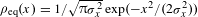, with variance σx2 = kBT/κ, as prescribed by the equipartition theorem. Here, kB is the Boltzmann constant and T the bath temperature. In this system, we consider the compression process sketched in Fig. 1, in which the stiffness is changed from an initial value to a larger one. The evolution of the system during the relaxation towards the new equilibrium state is monitored through the position pdf ρ(x, t), which is Gaussian at all times (see Methods and Supplementary Information). Thus, the distribution ρ(x, t) is fully characterized by the time evolution of its mean and its standard deviation σx(t). The main question is now that of finding, provided it exists, a suitable time evolution of the stiffness κ(t) (our control parameter), for which the equilibration process is much faster than τrelax. This question can be affirmatively answered using a particular solution of the Fokker–Planck equation (see Methods and Supplementary Information). We emphasize that the ESE idea is not restricted to manipulating Gaussian states, and that non-harmonic potentials U can be considered, along the lines presented in the Supplementary Information.

In this Letter, two methods are compared. On the one hand, at a given instant ti = 0, we suddenly change κ from the initial value κi to the final value κf. During this protocol, referred to as STEP, the particle mean position does not change, whereas the spread σx equilibrates to the new value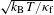in about 3 relaxation times τrelax = γ/κf. On the other hand, following the ESE procedure, κ(t) is modulated in such a way that σx is fully equilibrated at tf τrelax. The protocol which meets our requirements is given by equation (8) (in Methods). In the experiment, we select κi = 0.5 pN μm−1 and κf = 1.0 pN μm−1 in such a way that τrelax 15 ms. Furthermore, to have a well-defined separation between timescales, we choose tf = 0.5 ms, which is roughly 100 times smaller than the thermalization time in the STEP protocol. Both protocols are shown in Fig. 2a, where we can appreciate the rather complex time dependence of the ESE control procedure. This is a necessity to allow for a quick evolution to the new equilibrium state. The faster the evolution (smaller tf), the stiffer the transient confinement must be (the maximum stiffness reached in Fig. 2a is 37 κi). To study the evolution of ρ(x, t) for the two protocols, we perform the following cycle. First, the particle is kept at κi for 50 ms to ensure proper equilibration. Then, at t = 0 ms we apply the protocol (either STEP or ESE) and x(t) is measured for 10 ms in the case of ESE and 100 ms for STEP. Finally, the stiffness is set again to κi and this cycle is repeated N times. The evolution of σx(t) for t > 0 is obtained by performing an ensemble average over N = 2 × 104 cycles.Figure 2: Dynamics of the system along the STEP and ESE protocol.

The results are shown in Fig. 2b, where σx(t) is plotted as a function of time for the two protocols, from one equilibrium configuration to the other. It appears that the engineered system reaches the target spread precisely at tf, and subsequently does not evolve. On the other hand, the STEP equilibration occurs after a time close to 3τrelax. Figure 2c, d represents the complete STEP and ESE dynamics of ρ(x). The Gaussian feature is confirmed experimentally during ESE, even far from equilibrium, as the kurtosis is Kurt(x) = (3.00 ± 0.01). The results of Fig. 2 clearly show the efficiency of ESE, driving the system into equilibrium in a time which is 100 times shorter than the nominal equilibration time 3τrelax.

We now turn our attention to the energy required to achieve such a large time reduction. Developments in the field of stochastic thermodynamics29 endow work W and heat Q with a clear mesoscopic meaning, from which a resolution better than kBT can be achieved experimentally (see Methods for an explicit definition). In Fig. 3, the complete energetics of our system is shown for the ESE and STEP protocols. The evolution of the mean cumulative work 〈W(t)〉 reveals the physical behaviour of the system undergoing ESE. In the first part of the protocol (t < 0.2 ms), confinement is increased, which provides positive work to the system. In the subsequent evolution (0.2 < t < 0.5 ms), work is delivered from the system to the environment through the decrease of the stiffness. In striking contrast to an adiabatic transformation, the value of heat increases monotonically, as the system dissipates heat all over the protocol. In the inset of Fig. 3, 〈Q〉 and 〈W〉 are shown for the STEP process. Notice how the work exerted on the system is almost instantaneous, whereas heat is delivered over a wide interval of time, up to complete equilibration. As expected, there is a price to pay for ESE. A significant amount of work is required to speed up the evolution and beat the natural timescale of our system22. It can be shown that the cost 〈W(tf)〉 behaves like τrelax/tf for tf → 0. More precisely, this amounts to a time–energy uncertainty relation: tf 〈W(tf)〉 0.106 (2τrelax) kBT. If instead, one proceeds in a quasi-static fashion (tf τrelax), the cost reduces to the free energy difference, kBTlog(κf/κi)/2, which is 0.35 kBT when κf = 2κi. For the ESE experiments shown, we have 〈W(tf)〉 7.0 kBT, about twenty times larger.

Our results show the feasibility and expediency of accelerated protocols for equilibrating confined Brownian objects. The ESE path allows a gain of two orders of magnitude in the thermalization time, as compared to an abrupt change of control parameter (STEP process). The associated energetic cost has been assessed. Finally, although an overdamped problem has been solved here, the generalization of the ESE protocol to non-isothermal regimes for underdamped systems can in principle be worked out theoretically. Its application to AFM tips, vacuum optical traps, or to transitions between non-equilibrium steady states, constitutes a timely experimental challenge in this emerging field.

## Methods

### Experimental set-up.

Silica microspheres of radius 1 μm were diluted in milliQ water to a final concentration of a few spheres per millilitre. The microspheres were inserted into a fluid chamber, which can be displaced in three dimensions by a piezoelectric device (Nanomax TS MAX313/M). The trap is realized using a near-infrared laser beam (Lumics, λ = 980 nm with maximum power 500 mW) expanded and injected through an oil-immersed objective (Leica, 63 × NA 1.40) into the fluid chamber. The trapping laser power, which determines the trap stiffness, is modulated by an external voltage Vκ by means of a Thorlabs ITC 510 laser diode controller with a switching frequency of 200 kHz. Vκ is generated by a National Instrument card (NI PXIe-6663) managed by a custom-made Labview program. The detection of the particle position is performed using an additional HeNe laser beam (λ = 633 nm), which is expanded and collimated by a telescope and passed through the trapping objective. The forward-scattered detection beam is collected by a condenser (Leica, NA 0.53), and its back focal-plane field distribution projected onto a custom position sensitive detector (PSD from First Sensor with a bandpass of 257 kHz) whose signal is acquired at a sampling rate of 20 kHz with a NI PXIe-4492 acquisition board.

### Energetics measurement.

From the experimental observables, the stiffness κ and the particle position x, it is possible to infer the energetic evolution of our system within the stochastic energetics framework29. The notion of work W is related to the energy exchange stemming from the modification of a given control parameter—here the trap stiffness. Alternatively, heat Q pertains to the energy exchanged with the environment, either by dissipation or by Brownian fluctuations. The work W(t) and dissipated heat Q(t) are expressed as W(t) = ∫ 0t(∂U/∂κ)°(dκ/dt′)dt′, Q(t) = −∫ 0t(∂U/∂x)°(dx/dt′)dt′, where ° denotes the Stratonovich integral and U is the potential energy. Under this definition, the first law reads as ΔU(t) = W(t) − Q(t), where W(t), Q(t) and ΔU(t) are fluctuating quantities. Because T is fixed, both ESE and STEP processes share the same value 〈ΔU(tf)〉 = 0 between the initial and the final state. As a consequence, we have 〈W(tf)〉 = 〈Q(tf)〉.

### ESE protocol for the harmonic potential.

Although the idea is general (as discussed in Supplementary Information), we first start by a presentation applying the method to harmonic confinement. The dynamics of the system is then ruled by the Langevin equation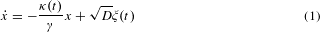where a dot denotes time derivative and x is for the position of the Brownian particle. The friction coefficient γ = 6πνR is here constant, ν being the kinetic viscosity coefficient and R the radius of the bead. The diffusion constant then reads as D = kBT/γ. The stiffness κ has an explicit dependence on time and ξ(t) is a white Gaussian noise with autocorrelation 〈ξ(t)ξ(t + t′)〉 = 2δ(t′). Equation (1) is overdamped (there is no acceleration term in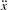), which is fully justified for colloidal objects30. The Langevin description equation (1) can be recast into the following Fokker–Planck equation for the probability density31: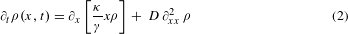At initial and final times (ti and tf), ρ(x, t) is Gaussian, as required by equilibrium. A remarkable feature of the ESE (non-equilibrium) solution is that, for intermediate times, ρ(x, t) remains Gaussian,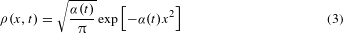We demand that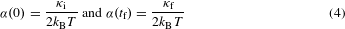Combining equation (2) with equation (3), we obtain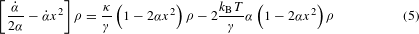Requiring that the equality holds for any position x, the equation is simplified into: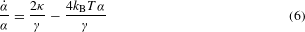This relation was obtained in refs 23,24 by studying the evolution of the variance σx2. However, unlike in these works, we supplement our description with the constraints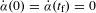, as a fingerprint of equilibrium for both t < 0 and t > tf.

Next, the strategy goes as follows. We choose the time evolution of α, complying with the above boundary conditions. To this end, a simple polynomial dependence of degree 3 is sufficient. Other more complicated choices are also possible. Introducing the rescaled time s = t/tf, we have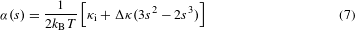where Δκ = κfκi. Finally, equation (6) has been satisfied, from which we infer the appropriate evolution κ(t) that is then implemented in the experiment: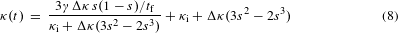The analysis, restricted here to the one-dimensional problem, can be easily recast in three dimensions. It is also straightforward to generalize the idea to account for a time-dependent temperature T(t), which can be realized experimentally20. In this latter situation, the key relation equation (6) is unaffected, and therefore indicates how κ should be chosen, for prescribed α(t) and T(t). This highlights the robustness of the ESE protocol.

The mean work exchanged in the course of the transformation takes a simple form in our context: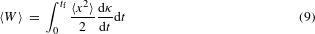According to our ansatz (3), 〈x2〉 = 1/(2α(t)), and using the relation (6), equation (9) can be written in the following form23,24: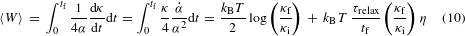where τrelax = γ/κf and η is a numerical factor given by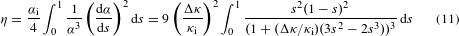Notice that equation (10) coincides with expressions derived in previous works32,33 using linear response theory.

For our parameters, we find η 0.106, as indicated in the main text. Interestingly, expression (10) gives the free energy difference value in the limit tf τrelax, 0.5kBTlog(κf/κi), which appears as the minimal mean work. In the opposite limit, we have a time–energy relation: tfW〉 = kBrelax(κf/κi)η. We emphasize that the scaling in 1/tf when tf → 0 is ansatz independent, although the specific value of the η parameter depends on the ansatz. It can be shown that the lowest η value for all admissible protocols is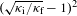, which gives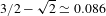here. Thus, our protocol, although sub-optimal in terms of mean work, nevertheless has an η value close to the best achievable.

### Data availability.

The data that support the plots within this paper and other findings of this study are available from the corresponding author on request.